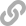Master of Finance

# Suggested Background

## Mathematical Background and Programing Skills• ### Linear algebra:Basic topics, including: matrix/vector notation, operations on matrices and vectors, determinants, eigenvalues and eigenvectors, quadratic forms, and systems of linear equations.

• ### Calculus:Multivariable differentiation and integration, series expansions, and function approximation and maximization.

• ### Probability:Sample spaces and random variables, common distributions and densities, moments of distributions, conditional probability and Bayes’ theorem, law of large numbers, central limit theorem, joint distributions, covariance, correlation, and stochastic independence.

• ### Stochastic processes:Random walks, Bernoulli trials, Markov processes, basic properties of linear time series models, continuous-time processes, and Ito’s lemma.

• ### Statistics/econometrics:Parameter estimation, confidence intervals, hypothesis tests, linear regression models, ordinary least squares, and likelihood principle.

• ### Computer literacy:Students entering the MIT MFin program are expected to possess basic programming skills needed for processing and analyzing data. As part of the degree requirements, all students are required to sit for and pass the Programming Literacy Test using any of the following programming languages: R or Python.

##### Self-assessment

To assess the adequacy of your mathematical background, please use the following self-assessment test. If you experience difficulties in any particular area, we strongly recommend that you strengthen your skills through self-study or formal coursework prior to enrolling in the MFin program.

## Self-study Resources• ### Programming:DataCamp is an online interactive training and education platform in the field of data science and programming.

Helpful resources to prepare for the PLT are the following:

-Ang, Analyzing Financial Data and Implementing Financial Models Using R

-Ruppert and Matteson, Statistics and Data Analysis for Financial Engineering

-Arratia, Computational Finance

• ### Mathematics:MIT OpenCourseWare provides access to many resources that may be helpful, including lecture videos, lecture notes, problem sets, exams, and solutions.

• 18.02 Multivariable Calculus (as taught in Fall 2007)*

–Lectures 1-4: some vector and matrix properties

–Lectures 8-13: partial derivatives; Lagrange multipliers

•18.06: Linear Algebra (as taught in 2010)*

•6.041: Probabilistic Systems Analysis and Applied Probability (as taught in 2010)*